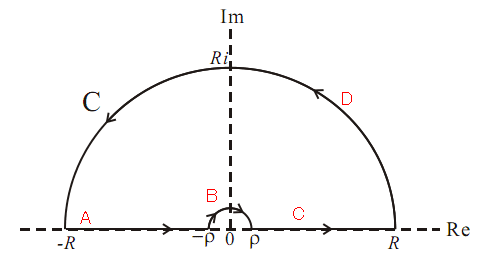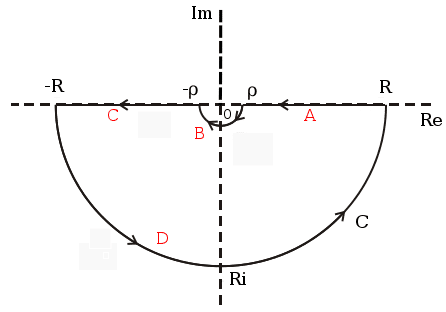# Fourier Transform of a Sinc function

A few days ago, I was trying to do the convolution between a Sinc function and a Gaussian function. But I got stuck from the first step, when I tried to solve that by using the convolution theorem, namely the Fourier transform of the Sinc(x), although I knew it is very easy to find the right answer by Googling or Mathematica. But it is worth a try to be done by hand. So, here we go:

We know that the Fourier transform of Sinc(z) is,andSo,

(1)Let us consider the first item, when, namely, we can choose the path below to do the contour integration.Using the method of complex residues, we take the contour with no singular point, separating the path into four parts, namely A, B, C and D shown as the red letters in the figure. Thus,

(2)Also if, namely, and whenand, it is easy to prove using Jordan’s lemma, that the fourth term is equal to zero. Thus we can the rewrite the rest items as,In order to calculate the value of the item on the right side, I use, so, then we have,So, in the end, we get the value of the first integration item shown in the Eq.(1),

(3)When, according to Jordan’s lemma, we can not get the fourth item in the Eq.(2) to be zero. So we have to choose another path for finalizing the integration.
So I chose the path below to deal with such a situation,It is not hard to calculate the same way as the previous one,

(4)and here again I use the same trick,, so. Because,So, in the end,

(5)Now, let’s move on to the second part in the Eq.(1). Following the same steps, using contour above real axis I get,

(6)and using contour blow real axis I get,

(7)Finally, we can write the answer as, ifand ifand(Note: if we define the Fourier Transform asThen the result could be a little different.)

1.Marvin says:

我发现我有很多的流量是从你这里来的~除了豆瓣就是这里了~

1.directus says:

友链？

2.赵小舟 says:

厉害，我已经不会用右手推公式了

1.directus says:

不厉害啊…我也忘光了。

3.Charles Kung says:

Thanks a lot !
正好在做这方面的作业
学习到了！

4.Charles Kung says:

不过想请教一下w=1(-1)时为什么不讨论啊

1.Directus says:

大概是因为不收敛吧。

This site uses Akismet to reduce spam. Learn how your comment data is processed.